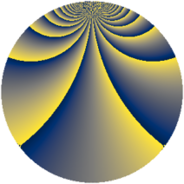# Properties

 Label 1152.3.zLevel $1152$ Weight $3$ Character orbit 1152.z Rep. character $\chi_{1152}(31,\cdot)$ Character field $\Q(\zeta_{12})$ Dimension $368$ Sturm bound $576$

# Learn more

## Defining parameters

 Level: $$N$$ $$=$$ $$1152 = 2^{7} \cdot 3^{2}$$ Weight: $$k$$ $$=$$ $$3$$ Character orbit: $$[\chi]$$ $$=$$ 1152.z (of order $$12$$ and degree $$4$$) Character conductor: $$\operatorname{cond}(\chi)$$ $$=$$ $$144$$ Character field: $$\Q(\zeta_{12})$$ Sturm bound: $$576$$

## Dimensions

The following table gives the dimensions of various subspaces of $$M_{3}(1152, [\chi])$$.

Total New Old
Modular forms 1600 400 1200
Cusp forms 1472 368 1104
Eisenstein series 128 32 96

## Trace form

 $$368q + 4q^{5} + O(q^{10})$$ $$368q + 4q^{5} + 4q^{13} - 32q^{17} - 28q^{21} + 4q^{29} - 16q^{33} + 16q^{37} + 108q^{45} - 960q^{49} + 16q^{53} + 4q^{61} - 8q^{65} - 28q^{69} - 388q^{77} - 16q^{81} + 104q^{85} - 52q^{93} - 8q^{97} + O(q^{100})$$

## Decomposition of $$S_{3}^{\mathrm{new}}(1152, [\chi])$$ into newform subspaces

The newforms in this space have not yet been added to the LMFDB.

## Decomposition of $$S_{3}^{\mathrm{old}}(1152, [\chi])$$ into lower level spaces

$$S_{3}^{\mathrm{old}}(1152, [\chi]) \cong$$ $$S_{3}^{\mathrm{new}}(144, [\chi])$$$$^{\oplus 4}$$$$\oplus$$$$S_{3}^{\mathrm{new}}(576, [\chi])$$$$^{\oplus 2}$$